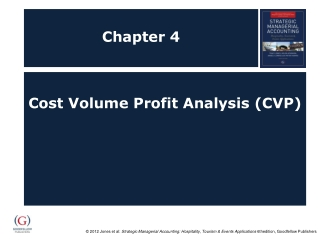DownloadDownload PresentationChapter 4

Chapter 4

Télécharger la présentationChapter 4

- - - - - - - - - - - - - - - - - - - - - - - - - - - E N D - - - - - - - - - - - - - - - - - - - - - - - - - - -
Presentation Transcript

1. Chapter 4 Cost Volume Profit Analysis (CVP)

2. Objectives After studying this topic you should be able to: Understand the concept and terminology of CVP; Have a working knowledge of CVP calculations; Appreciate the usefulness of CVP to managers as a decision making tool; and Explore the use of ‘What if?’ case scenarios with the aid of computer spread sheets.

3. CVP terminology and concept (part 1) Profit = Total Sales Revenue – Total costs Contribution margin = Sales Revenue – Variable costs Selling price – variable costs per unit = contribution per unit Breakeven point (BEP) in units = Fixed Costs/contribution per unit BEP for required profit = Fixed Costs + Required Profit Contribution per unit

4. CVP terminology and concept(part 2) C/S ratio = Contribution margin * 100 Sales Revenue BEP in £ sales revenue = Fixed costs C/S ratio Margin of Safety, in units = Estimated sales – breakeven sales Margin of Safety % = Estimated sales – breakeven sales *100 Estimated sales

5. Contribution Margin profit statement for Oriental Delight Restaurant Example

6. Solution (part 1) Selling price – variable costs per unit = contribution per unit £10 - £4 = £6 contribution per unit Breakeven point (BEP) in units = Fixed Costs/contribution per unit £120,000/£6 = 20,000 units To make a £15000 profit:£120,000 + £15,000 = 22,500 units £6 C/S ratio = Contribution margin * 100 Sales Revenue £90,000 * 100 = 60% £150,000

7. Solution (part 2) Oriental Delight BEP = £120,000 = £200,000 0.60 (60%) Margin of Safety, in units = Estimated sales – breakeven sales Where estimated sales are 25000 units 25,000 – 20,000 = 5,000 units Margin of Safety % 25,000 – 20,000 *100 = 20% 25,000

8. Graphical representations of CVP/BEP

9. Margin of Safety on Graph

10. Contribution Chart

11. Profit/Volume Chart

12. Underlying assumption in CVP analysis Revenue and cost behaviour are linear i.e. are straight lines on the chart; All variables, other than those under consideration remain constant, such as Selling price per unit will not change Variable costs vary in direct proportion to sales Fixed costs remain constant Cost prices remain constant e.g. food costs and staff wage rates Productivity remains unchanged Methods of production and service remain unchanged It covers a single product, or if multiple products (menu items) the sales mix remains unchanged; All costs can be segregated into their fixed and variable components; Revenue and costs are related to a single independent variable, which is level of activity (units sold); and Volume of activity is the only relevant variable that determines cost behaviour.

13. Computerised ‘What if?’ analysis Benefits include: Allows rapid computation Gives instant feedback Provides data flexibility Provides alternative document forms Formula can be viewed

14. Spreadsheet design guidelines Prepare initial design on paper Separate input and output areas Enter a decision variable only once Never include a decision variable in a formula Include a summary output screen Incorporate instructions for users Test the spreadsheet